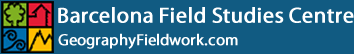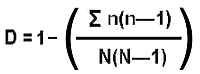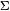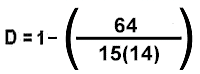# Simpson's Diversity Index

A community dominated by one or two species is considered to be less diverse than one in which several different species have a similar abundance.

Simpson's Diversity Index is a measure of diversity which takes into account the number of species present, as well as the relative abundance of each species. As species richness and evenness increase, so diversity increases.n = the total number of organisms of a particular species N = the total number of organisms of all species

The value of D ranges between 0 and 1. With this index, 1 represents infinite diversity and 0, no diversity.

To calculate Simpson's Index for Muntanyans, two areas (natural vegetation and disturbed vegetation) must be sampled using quadrats placed randomly or systematically. The number of plant species within each quadrat, as well as the number of individuals of each species should be noted. There is no necessity to be able to identify all the species, provided they can be distinguished from each other.

As an example, let us work out the value of D for a single quadrat sample of ground vegetation in the Muntanyans dunes. Of course, sampling only one quadrat would not give you a reliable estimate of the diversity of the dune flora. Several samples would have to be taken and the data pooled to give a better estimate of overall diversity. The method used to optimise the sampling is the Optimum Quadrat Size technique.

Species Number (n) n(n-1)
Sea holly 2 2
Sand couch 8 56
Sea bindweed 1 0
Sporobolus pungens 1 0
Echinophora spinosa 3 6
Total 15 64
 N = 15n(n-1) = 64

Putting the figures into the formula for Simpson's Index:Simpson's Index of Diversity = 0.7